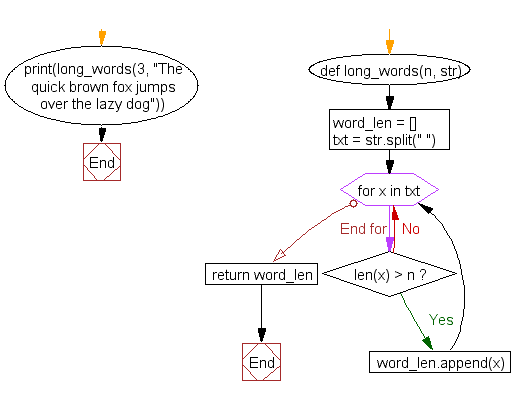﻿ Python: Find the list of words that are longer than n from a given list of words - w3resource

# Python: Find the list of words that are longer than n from a given list of words

## Python List: Exercise-10 with Solution

Write a Python program to find the list of words that are longer than n from a given list of words.

Pictorial Presentation:Sample Solution:

Python Code:

``````def long_words(n, str):
word_len = []
txt = str.split(" ")
for x in txt:
if len(x) > n:
word_len.append(x)
return word_len
print(long_words(3, "The quick brown fox jumps over the lazy dog"))
```
```

Sample Output:

```['quick', 'brown', 'jumps', 'over', 'lazy']
```

Explanation:

In the above exercise -

def long_words(n, str):  -> Defines a function called ‘long_words’ that takes two parameters: 'n' and 'str'. 'n' is an integer that represents the minimum length of words to be returned by the function, and 'str' is a string that will be processed by the function.

word_len = []  -> Initialize an empty list called 'word_len'.

txt = str.split(" ")  -> This line splits the input string 'str' into a list of words using the split() method and stores in the 'txt' variable. The argument " " passed to split() specifies that words in the string should be separated by spaces.

```for x in txt:
if len(x) > n:
word_len.append(x)```

The above lines loop through each word in the 'txt' list and check if its length is greater than n. If the length of the word is greater than n, the word is added to the word_len list using the append() method.

return word_len  -> This line returns the word_len list, which contains all the words in the input string that have a length greater than n.

print(long_words(3, "The quick brown fox jumps over the lazy dog"))  -> This line calls the 'long_words' function with the input parameters 3 and "The quick brown fox jumps over the lazy dog". The function returns a list of all the words in the input string that have a length greater than 3.

Finally output will be ['quick', 'brown', 'jumps', 'over', 'lazy']

Flowchart:## Visualize Python code execution:

The following tool visualize what the computer is doing step-by-step as it executes the said program:

Python Code Editor:

Have another way to solve this solution? Contribute your code (and comments) through Disqus.

What is the difficulty level of this exercise?

Test your Programming skills with w3resource's quiz.

﻿

## Python: Tips of the Day

Given a predicate function, fn, and a prop string, this curried function will then take an object to inspect by calling the property and passing it to the predicate:

Example:

```def tips_check_prop(fn, prop):
return lambda obj: fn(obj[prop])
check_age = tips_check_prop(lambda x: x >= 25, 'age')
user = {'name': 'Owen', 'age': 25}

print(check_age(user))
```

Output:

```True
```

We are closing our Disqus commenting system for some maintenanace issues. You may write to us at reach[at]yahoo[dot]com or visit us at Facebook